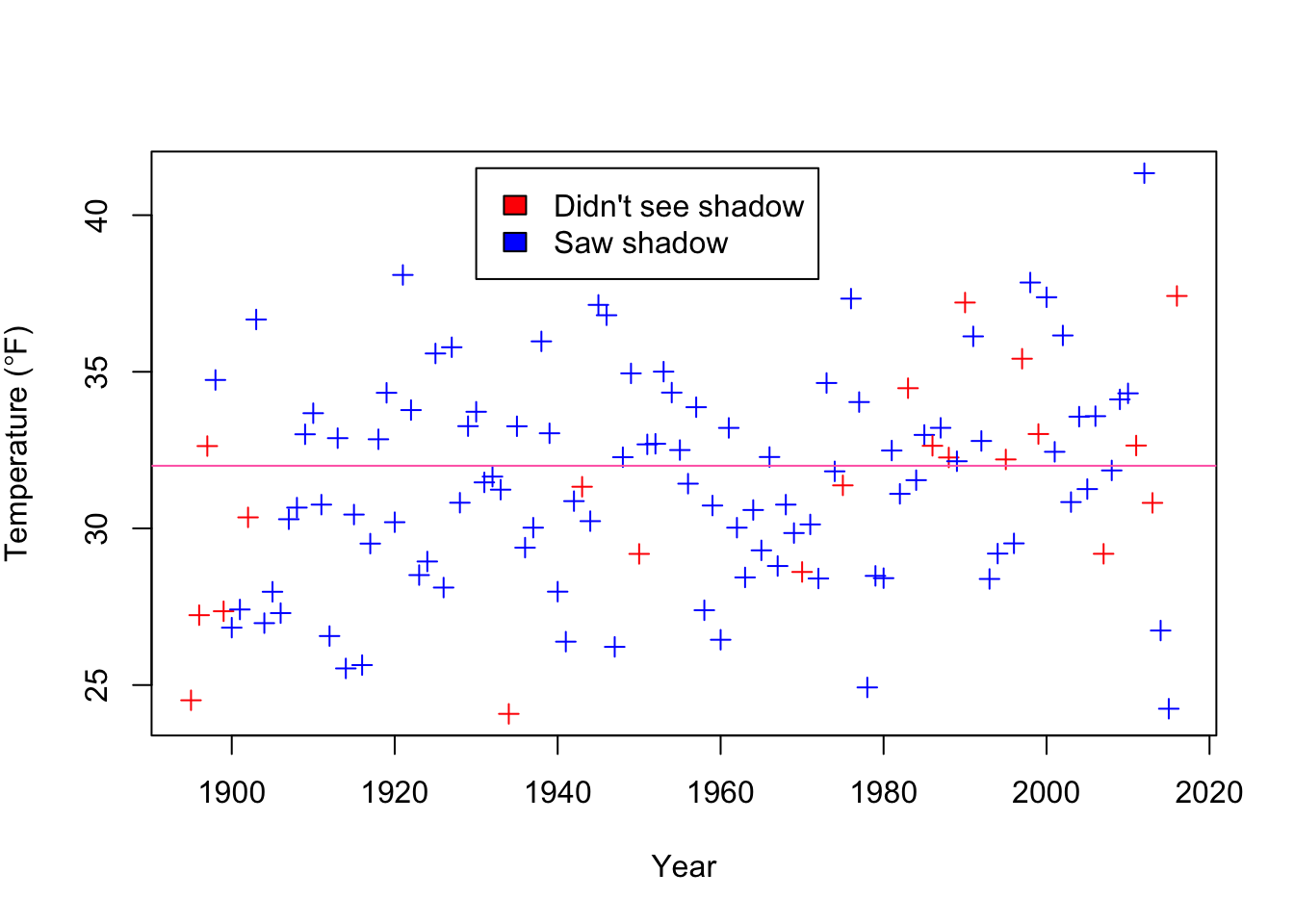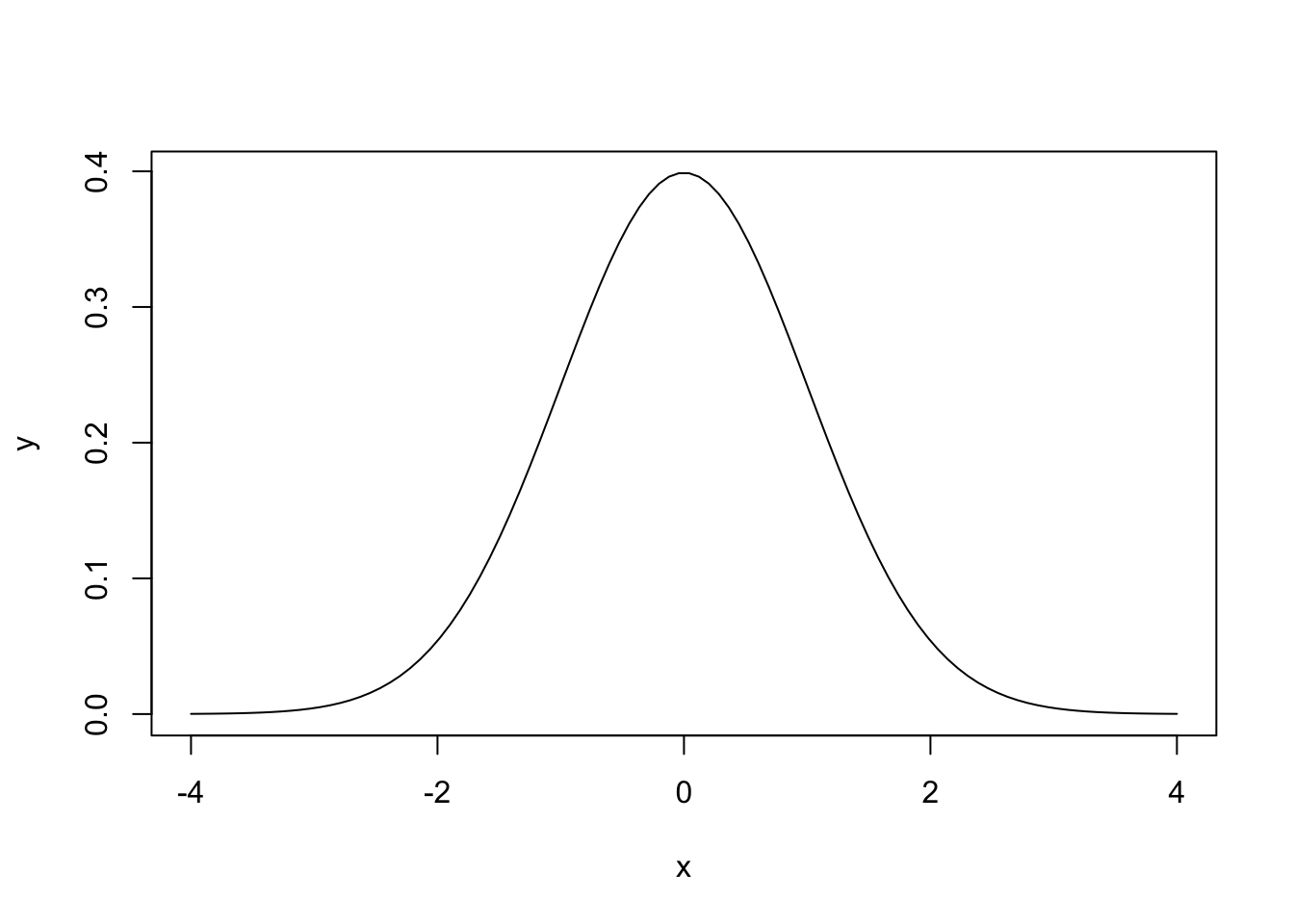## Recap

So far we have

• learned about maximising the likelihood
• estimated confidence intervals and standard errors

These are the basic tools we will use to fit and understand our models

## More than one datum

So far we have only used one data point. But what if we have more? The standard approach starts by making one additional assumption: that each data point is collected independently of the rest. This would mean that each time data is collected, it doesn’t depend on what the last data point was.

If we assume that the data are independent, then from the definition of independence:

$$Pr(X_1 \& X_2) = Pr(X_1) Pr(X_2)$$

So, because the likelihood is the probability of each data point given the parameters, we can calculate the likelihood for the parameters ($$\theta$$) given all of the data ($$X_i$$’s) by multiplying the probabilities:

$Pr(X_1, X_2,...,X_n|\theta) = Pr(X_1|\theta)Pr(X_2|\theta)...Pr(X_n|\theta) = \prod_ {i=1}^{n} Pr(X_i\theta)$

But we usually work on the log-likelihood scale, where we have:

$l(\theta|X_1, X_2,...,X_n) = \sum_{i=1}^{n} \log(Pr(X_i|\theta))$

So, we just add the log-likelihoods together, which is easier than multiplying, and makes a lot of the maths easier.

## Punxsutawney Phil & Groundhog Day

Every 2nd of February, the town of Punxsutawney in the US has a ritual where they go to Gobbler’s Knob, drag a groundhog out of the ground, call it Phil, and ask it if it sees Bill Murray’s its shadow. If Punxsutawney Phil sees his shadow, he’ll decide to retreat, because there will be another 6 weeks of winter.

The question is whether Punxsutawney Phil is actually any good at predicting the weather. We will answer that by looking at whether he sees his shadow, and the temperature for the following 6 weeks.

GDay <- read.csv(file="https://www.math.ntnu.no/emner/ST2304/2019v/Week4/GroundhogDayta.csv")

plot(GDay$Year, GDay$Temperature, cex=1, pch=3,
xlab="Year", ylab = expression(paste("Temperature (",degree,"F)")))
legend(1930, 41.5, c("Didn't see shadow", "Saw shadow"), fill=c("red", "blue"))
abline(h=32, col="hotpink")Before getting to the question of whether Phil is any good as a weatherman, we’ll look at the distribution of the temperature. Of the 122 observations, 56 are above freezing. But we want to summarise the data in more detail, partly so we can ask more complicated questions and also so we can develop the theory. We will do this with the normal distribution.

## The Normal Distribution

The normal distribution looks like this:Or, for those who like equations, like this:

$f(x| \mu, \sigma^2) = \frac{1}{\sqrt{2 \pi \sigma^2}} e^{-\frac{(x - \mu)^2}{2\sigma^2} }$ $$x$$ is the thing that follows a normal distribution (e.g. temperature). $$f(x|\mu, \sigma^2)$$ is the density: a higher density means that value of $$x$$ is more likely1. We call $$f(x|\mu, \sigma^2)$$ the probability density function, and use it to calculate the probability that $$x$$ is between two values (e.g. $$Pr(-1.96 < x < 1.96| \mu=0, \sigma^2 = 1) = 0.95$$ ) The parameters are $$\mu$$ and $$\sigma^2$$: the mean and variance (sometikmes we use the standard deviation, $$\sigma$$, rather than $$\sigma^2$$, or even $$1/\sigma^2$$, the precision).

We can split the function up into bits. The first part is

$\frac{1}{\sqrt{2 \pi \sigma^2}}$

which does not depend on $$x$$, so is a constant. It is there because the area under the whole curve has to equal 1. More important is the second part

$e^{-\frac{(x - \mu)^2}{2\sigma^2} }$

If we take the log transformation (so we have the log probability, or later the log likelihood) we see that it is this

$-\frac{(x - \mu)^2}{2\sigma^2}$

So is a quadratic curve, and looks like this: